TAG: Equation Solving
March 19, 2010–

Steven Strogatz, a professor of applied mathematics at Cornell University, is currently blogging for The New York Times about issues “from the basics of math to the baffling”. It’s been a fascinating series, starting with preschool math and progressing through subtraction, division, complex numbers, and more. As Wolfram|Alpha is such a powerful tool for working with mathematical concepts, we thought it’d be fun to show how to use it to explore some of the topics in Strogatz’s blog.

First up is Strogatz’s post on “Finding Your Roots”. For a brief introduction to Wolfram|Alpha’s ability to find roots, try “root of 4x+2”.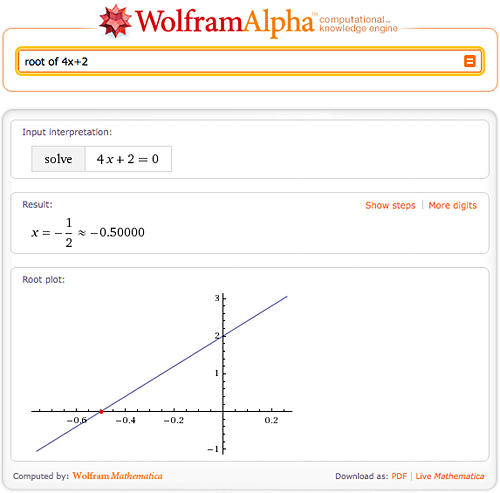Here we found the one and only root of 4x+2, but what if there is more than one root? Not a problem for Wolfram|Alpha—try “4x^2 + 3x – 4”. More »

January 14, 2010–

Need a tutor for solving equations? Solving equations is just one of hundreds of mathematical tasks that can be done using Wolfram|Alpha. Wolfram|Alpha can solve equations from middle school level all the way through college level and beyond. So next time you are stumped on an equation, consult Wolfram|Alpha for a little help.

Let’s start with the simpler stuff. Wolfram|Alpha can easily solve linear and quadratic equations, and even allows you to view a step-by-step solution of each problem.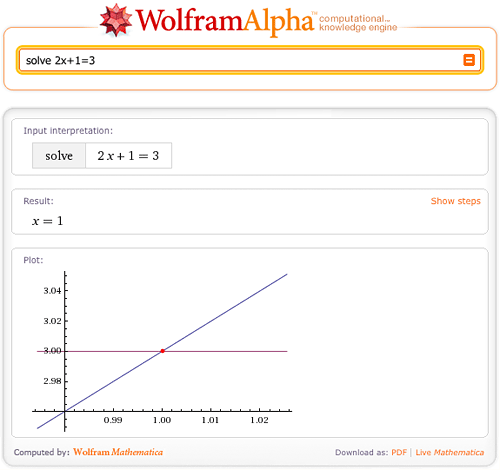What if the roots of the equation are complex? No worries; Wolfram|Alpha has no trouble solving equations over the complex plane.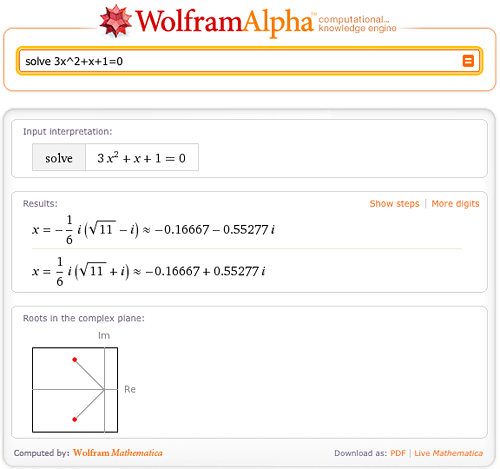Wolfram|Alpha can also solve cubic and quartic equations in terms of radicals.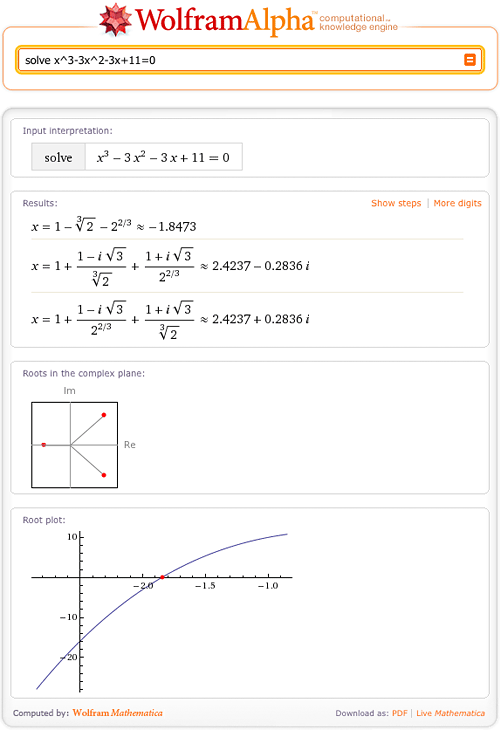Of course, some solutions are too large or cannot be represented in terms of radicals; Wolfram|Alpha will then return numerical solutions with a “More digits” button. More »

December 1, 2009–

## July 17, 2019 Update: Step-by-step solutions has been updated! Learn more.

Have you ever given up working on a math problem because you couldn’t figure out the next step? Wolfram|Alpha can guide you step by step through the process of solving many mathematical problems, from solving a simple quadratic equation to taking the integral of a complex function.

When trying to find the roots of 3x2+x–7=4x, Wolfram|Alpha can break down the steps for you if you click the “Show steps” button in the Result pod.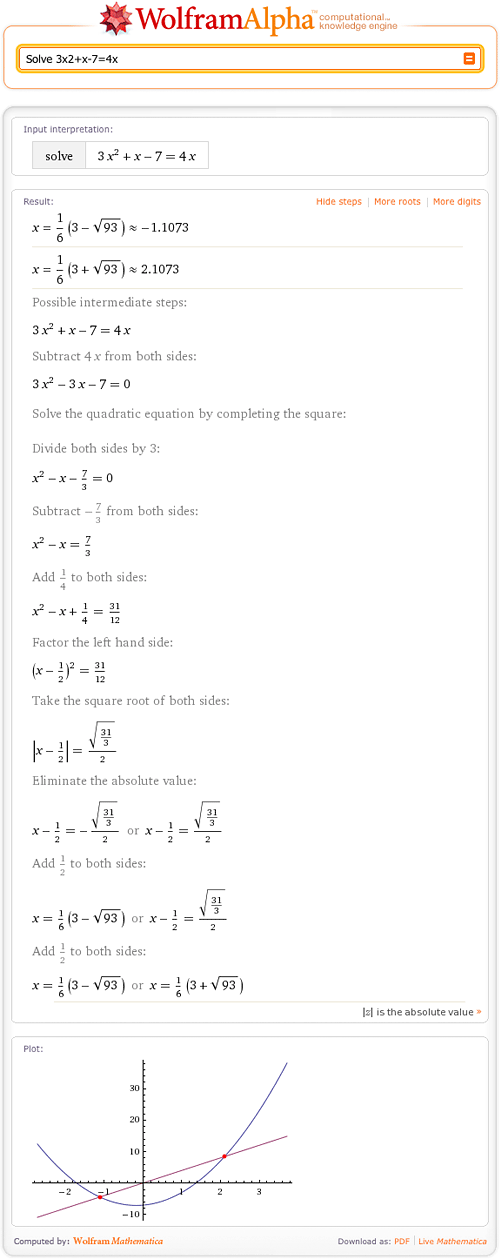As you can see, Wolfram|Alpha can find the roots of quadratic equations. Wolfram|Alpha shows how to solve this equation by completing the square and then solving for x. Of course, there are other ways to solve this problem! More »

September 3, 2009–

We use this blog to provide helpful tips on using Wolfram|Alpha. So when a relevant screencast caught our eye on Twitter—“Wolfram|Alpha for Calculus Students,” produced by Robert Talbert, PhD, an associate professor of mathematics and computing science at Franklin College—we wanted share it with you. We think his straightforward video is a great demonstration of just how valuable Wolfram|Alpha is for students. In the screencast, Professor Talbert discusses the concept of Wolfram|Alpha, and illustrates how it solves problems such as factoring or expanding expressions, solving quadratic equations, and more.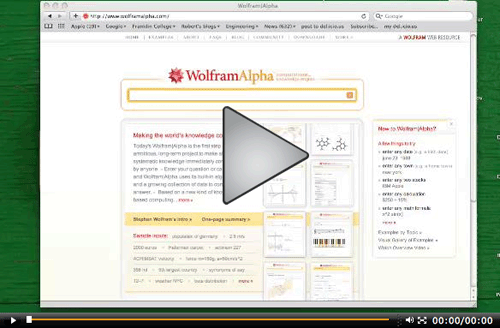The screencast covers just a few of the ways educators and students are using Wolfram|Alpha. Are you an instructor who has found innovative ways to incorporate Wolfram|Alpha into your lesson plans? Or are you a student using Wolfram|Alpha to assist in your studies? You can join others having these conversations on the Wolfram|Alpha Community site.